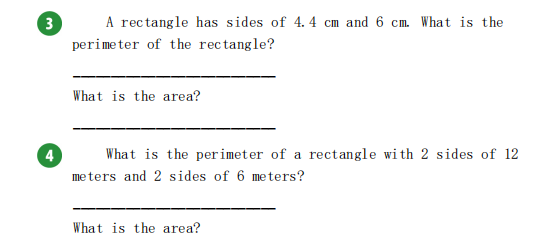### Still have math questions?

Arithmetic
Question(3) A rectangle has sides of $$4.4 cm$$ and $$6 cm$$ . What is the perimeter of the rectangle? What is the area?

(4) What is the perimeter of a rectangle with $$2$$ sides of $$12$$ meters and $$2$$ sides of $$6$$ meters? What is the area?

3.

Perimeter: $$P = 20.8\space cm$$

Area: $$A= 26.4\space cm^2$$

4.

Perimeter: $$P = 36\space m$$

Area: $$A = 72\space m^2$$

Solution
View full explanation on CameraMath App.